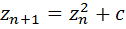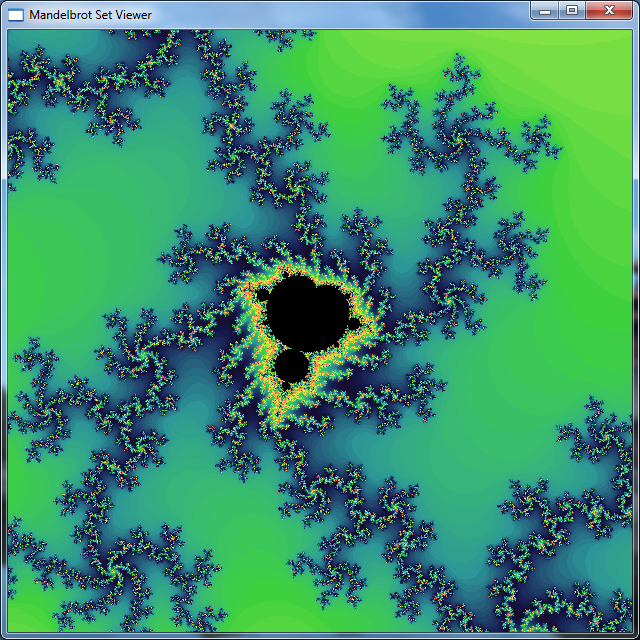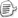C++ AMP全名C++ Accelerated Massive Parallelism（加速大规模并行计算）。是微软提出的基于C++的异构化并行计算平台。它将随Visual Studio 11一起发布，目前为预览版本。所谓异构并行计算，主要的需求就来自于GPU通用计算的崛起。GPU非常适合大规模数据并行算法，即同一程序应多多组不同的数据进行并行运算。然而GPU的架构与主流CPU不同，而且常常更新换代，没法采用传统编程语言来编程。现有的GPU多数编程方案，如DirectCompute和OpenCL，都要使用不同的语言或编译器来编写运行于GPU上的kernel部分和运行在CPU上的host部分。C++ AMP统一了这两部分，可以用同一个编译器，同一种语法来编写kernel代码；无需任何编译器选项或设置。更甚至C++ AMP的API简单到了极致，比OpenCL的方便程度更上了一个层次。下面我们就用C++ AMP来实现经典的曼德勃罗特集图形绘制。int a = 100, b = 100; array buffer(a, b);

 int a = 100, b = 100; std::vector myarray(a * b); array_view view(a, b, myarray);

 std::vector arr1(10); std::vector arr2(10); array_view view1(10, arr1); array_view view2(10, arr2); array r(10); parallel_for_each(r.grid, [view1, view2, &r](index<1> i) restrict(direct3d) { r[i] = view1[i] + view2[i]; });

 #include "amp.h" //#define FP64 #if defined FP64 #define _F(x) x typedef double fp_t; #else #define _F(x) x##f typedef float fp_t; #endif void generate_mandelbrot( Concurrency::array_view result, unsigned int max_iter, fp_t real_min, fp_t imag_min, fp_t real_max, fp_t imag_max );

 #include "stdafx.h" #include "mandelbrot.h" using namespace Concurrency; unsigned int set_hsb (float hue, float saturate, float bright) restrict (direct3d); void generate_mandelbrot( array_view result, unsigned int max_iter, fp_t real_min, fp_t imag_min, fp_t real_max, fp_t imag_max ) { int width = result.extent.get_x(); int height = result.extent.get_y(); fp_t scale_real = (real_max - real_min) / width; fp_t scale_imag = (imag_max - imag_min) / height; parallel_for_each(result.grid, [=](index<2> i) restrict(direct3d) { int gx = i.get_x(); int gy = i.get_y(); fp_t cx = real_min + gx * scale_real; fp_t cy = imag_min + (height - gy) * scale_imag; fp_t zx = _F(0.0); fp_t zy = _F(0.0); fp_t temp; fp_t length_sqr; unsigned int count = 0; do { count++; temp = zx * zx - zy * zy + cx; zy = 2 * zx * zy + cy; zx = temp; length_sqr = zx * zx + zy * zy; } while((length_sqr < _F(4.0)) && (count < max_iter)); //faster using multiplication than division float n = count * 0.0078125f; // n = count / 128.0f; float h = 1.0f - 2.0f * fabs(0.5f - n + floor(n)); //turn points at maximum iteration to black float bfactor = direct3d::clamp((float)(max_iter - count), 0.0f, 1.0f); result[i] = set_hsb(h, 0.7f, (1.0f - h * h * 0.83f) * bfactor); }); } unsigned int set_hsb (float hue, float saturate, float bright) restrict (direct3d) { float red, green, blue; float h = (hue * 256) / 60; float p = bright * (1 - saturate); float q = bright * (1 - saturate * (h - (int)h)); float t = bright * (1 - saturate * (1 - (h - (int)h))); switch ((int)h) { case 0: red = bright, green = t, blue = p; break; case 1: red = q, green = bright, blue = p; break; case 2: red = p, green = bright, blue = t; break; case 3: red = p, green = q, blue = bright; break; case 4: red = t, green = p, blue = bright; break; case 5: case 6: red = bright, green = p, blue = q; break; } unsigned int ired, igreen, iblue; ired = (unsigned int)(red * 255.0f); igreen = (unsigned int)(green * 255.0f); iblue = (unsigned int)(blue * 255.0f); return 0xff000000 | (ired << 16) | (igreen << 8) | iblue; }

C++ AMP的parallel_for_each调用是一种“准同步”的调用，当parallel_for_each完成时，GPU计算并不一定结束了，但代码会继续执行。只有当你访问计算的结果——比如包含结果的array_view时，它就会阻塞线程等待GPU计算的完成。我们可以调用array_view的synchornize方法来显式地等待。C++ AMP也提供真正异步接口，但用起来比较麻烦，在这个例子中就不采用了。下面的代码就是调用以及同步的代码。这里展示代码将结果保存在一个位图中：

 int _tmain(int argc, _TCHAR* argv[]) { GdiplusStartupInput gdiplusStartupInput; ULONG_PTR gdiplusToken; GdiplusStartup(&gdiplusToken, &gdiplusStartupInput, nullptr); const int edge = 1024; std::vector a(edge * edge); array_view av(edge, edge, a); generate_mandelbrot(av, 512, -2, -2, 2, 2); av.synchronize(); //construct a image { Bitmap output(edge, edge, edge * 4, PixelFormat32bppARGB, reinterpret_cast(a.data())); CLSID pngClsid; GetEncoderClsid(L"image/png", &pngClsid); output.Save(L"test.png", &pngClsid, nullptr); } GdiplusShutdown(gdiplusToken); return 0; }1. Windows 7 （不支持XP和Vista）

2. 硬件支持DirectX 11所有特性的显卡。如Nvidia Geforce 400,500系列显卡，AMD Radeon HD5000,6000,7000系列显卡。无法运行于不支持DirectX 11的显卡上。

3. 需要Visual Studio 11 Developer Preview。下载地址

4. 需要Windows SDK。下载地址posted on 2012-01-03 15:02  装配脑袋  阅读(10766)  评论(11编辑  收藏  举报